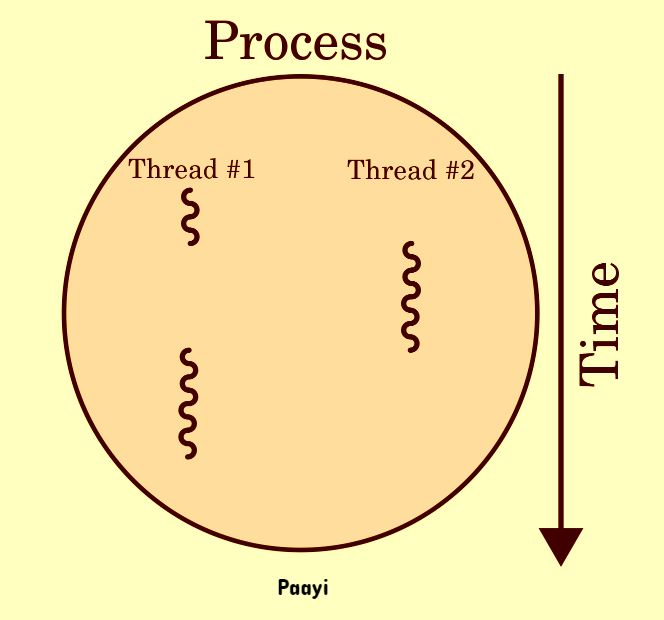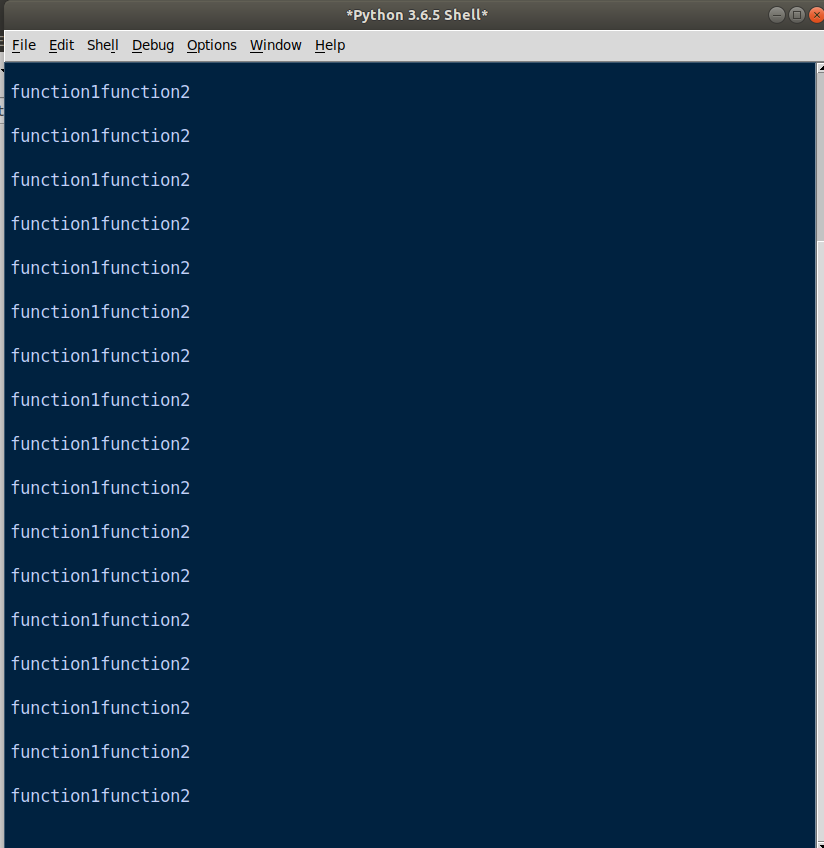## Python Tutorials: Learn Python Parallel Processing in Python

##### Python Tutorials: Learn Python Parallel Computing with Real Life Examples
Written by |19-Oct-2020 | 0 Comments | 780 Views

Parallel computing is a type of computing in which many functions are run simultaneously without blocking each other. Large problems are often divided into subproblems and then can be computed parallel so that users don’t have to wait to execute one program to start the other program. In our regular computer, there are multiple cores which perform and process multiple functions at a time so that user don’t have to use only one program at a time. This phenomenon is also called multitasking, which means doing multiple tasks at one time.

In programming languages, this can be achieved by multithreading and multiprocessing. On the web, there is an ajax call, which is the asynchronous call that executes the action without blocking the main thread; that is why you don’t have to wait for the execution to be done after clicking a button.

In this section, we will focus on multithreading and multiprocessing in python.Figure 1

Figure 1 shown above explains that multithreading is a technique of creating multiple tasks within the same process. Threads can communicate with each other in multithreading and coordinate.

Suppose we have two functions, and both of the function loops from 0 to 20 and print function1 and function2 on each iteration, respectively. The standard way of programming means synchronous programming will not start function 2 until function 1 is executed as follows:

 def func1():     for i in range (20):         print("function1")   def func2():     for i in range (20):         print("function2")   func1() func2()

If we run the above code, func2() will execute after func1() is executed. However, we can make it asynchronous to run parallel by multithreading as follows:

Now, if we run the above code, the output will be one after the other. There will be no block of code, and both will run asynchronously just like this:Running several threads at the same time is similar to running multiple programs at one time. Multiple threads within the same process share the information and can also communicate with each other. Threads are also called lightweight processes, and memory requirement is also very low.

In python3 we can use _thread and threading the 2 modules, but it is advised to used threading as _thread is the older and depreciated version. We can also use threading within a class. Threading can only be used with function and with the class, not with the following code. Following way is used in threading with the class:

The output for the above program is shown below.

The above example is using thread in classes. We first inherit the threading module into our class, and we initialize the threading instance in init. Then in a run function, we make a forloop from 0 to 5. Then we make 2 threads and execute both run parallel without blocking to each other. Following is the description of the built-in function that we have used previously.

Start (): The start method starts the new thread by calling the run method. It is to be noted here we have to make a run function in our class to start a thread; otherwise, the start () function will not recognize which function to call.

Join (): The join function will wait for the function to completely execute, and then it will terminate the function.

Multiprocessing:

Multiprocessing uses different memory space and multiple CPU cores. That is why multiprocessing is faster than multithreading. However, the coding style is approximately the same as we have done in multithreading.

In multiprocessing, there is no communication between the two processes. The worked independently without any coordination. Similar to multithreading, they can be used along with the function and classes. We cannot use it with synchronous code. The following method is used for the execution of the function:

 import multiprocessing def func1(num):     for i in range(num):         print (f"Function is: func1")   def func2(num):     for i in range(num):         print (f"Function is: func2")   if __name__ == "__main__":     iteration=1000       p1 = multiprocessing.Process(target=func1, args=(iteration,))     p2 = multiprocessing.Process(target=func2, args=(iteration,))       p1.start()     p2.start()       p1.join()     p2.join()

When you run in the idle, this function will not be executed because the multiprocessing function can only be executed in the terminal. Outside the terminal, it will show no output.

Pool Process:

By the pool process, we can create the number of workers in multiprocessing, which are the number of processes. By this procedure, we can pass multiple values at a time that will be executed concurrently. The following code is the practical application of the poll process in python:

 from multiprocessing import Pool def func(num):     return num**4     p = Pool (5) print (p.map (func, [1, 2, 3,4,5,6,7]))

The above code is a function that converts the number to rise to the power of 4. Here we have given 7 numbers in the list which are given to the function without making any for a loop. If we run this program in a terminal, we will get the following output:

 Output [1, 16, 81, 256, 625, 1296, 2401]

It is not necessary to write if __name__ == '__main__': in multiprocessing. It is actually a convention to write, but bot compulsory; without it, the code will work fine.

This was the introduction of multithreading and multiprocessing. Nowadays, these modules are not used vastly due to the presence of an efficient application program interface. We will see at a higher level what are the new modules and what is the disadvantage of using these modules till than practice on multithreading and multiprocessing to know the core of these two modules.